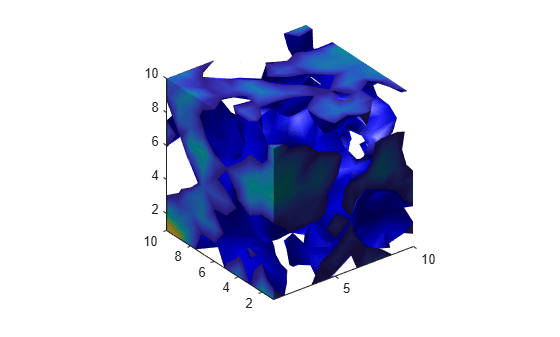smooth3

Smooth 3-D data

Description

W = smooth3(V) smooths the input data V and returns the smoothed data in W.

W = smooth3(V,'filter') filter determines the convolution kernel and can be one of these values:

• 'gaussian'

• 'box' (default)

W = smooth3(V,'filter',size) sets the size of the convolution kernel (default is [3 3 3]). If size is scalar, then size is interpreted as [size, size, size].

W = smooth3(V,'filter',size,sd) sets an attribute of the convolution kernel. When filter is gaussian, sd is the standard deviation (default is .65).

Examples

collapse all

Create a 10-by-10-by-10 array of random data and smooth it.

data = rand(10,10,10);
data = smooth3(data,'box',5);

Display the data as an isosurface with end caps.

patch(isocaps(data,.5),...
'FaceColor','interp','EdgeColor','none');
p1 = patch(isosurface(data,.5),...
'FaceColor','blue','EdgeColor','none');
isonormals(data,p1);
view(3);
axis vis3d tight
camlight left
lighting gouraud